Question

Write a program that will loop ten times. In each iteration prompt user to enter an integer between -50&50. Print the input, keep a running sum of the inputs, and track the minimun and maximum numbers input. After the loop is complete, minimum number entered, maximum number entered, sum of all numberes entered, and average of all numbers entered.

Format all output. Before running the loop, print the label "Input values: ". Within the loop, print value entered by user followed by a comma and a space (", ") unless it is the last input. In that case print a newline instead.

Print remaining values on their own line providing a formatted label for each one. All values should use ten spaces and be right justified. This assignment of for my computer science 121 class and it needs to be in C. I am totally lost on this one. Any suggestions would be greatly appreciated!!!!

#include <stdio.h>

int main(void) {

int i,n,sum,max,min;

sum = 0;

//assign max and min to -50 and 50 ,allowed range

max = -50;

min = 50;

printf("\nInput Values : ");

printf("\n");

for(i=1;i<=10;i++)

{

scanf("%d",&n);

if(i <10)

printf("%10d, ",n); //print , between numbers

else

printf("%10d\n",n); // no comma , print new line

sum = sum + n; //running sum

if(n > max)

max = n;

if(n < min)

min = n;

}

printf("\nThe maximum number is %d",max);

printf("\nThe minimum number is %d",min);

printf("\nSum of all numbers is %d",sum);

printf("\nThe average of all numbers is %d",sum/10);

return 0;

}

output:

```Input Values : 45 23 11 46 49 -43 -44 -1 -36 33
45,         23,         11,         46,         49,        -43,        -44,         -1,        -36,         33

The maximum number is 49
The minimum number is -44
Sum of all numbers is 83
The average of all numbers is 8
```

#### Earn Coins

Coins can be redeemed for fabulous gifts.

Similar Homework Help Questions
• ### C++ Program Write a do-while loop that continues to prompt a user to enter a number...

C++ Program Write a do-while loop that continues to prompt a user to enter a number less than 100, until the entered number is actually less than 100. End each prompt with a newline. Ex: For the user input 123, 395, 25, the expected output is: Enter a number (<100): Enter a number (<100): Enter a number (<100): Your number < 100 is: 25 #include <iostream> using namespace std; int main() { int userInput; /* Your solution goes here */...

• ### In Java please: Write a program that will prompt the user to enter GPA values one...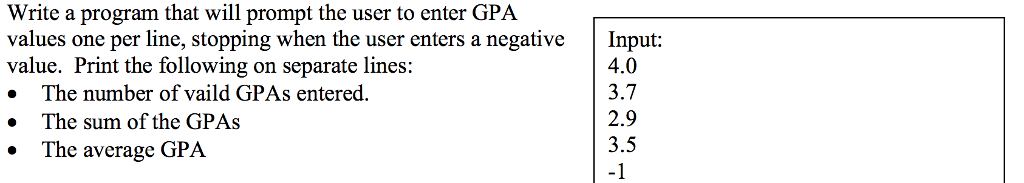In Java please: Write a program that will prompt the user to enter GPA values one per line, stopping when the user enters a negative Input: value. Print the following on separate lines: . The number of vaild GPAs entered » The sum of the GPAs 4.0 3.7 2.9 3.5 -1 The average GPA

• ### Write a do-while loop that continues to prompt a user to enter a number less than...

Write a do-while loop that continues to prompt a user to enter a number less than 100, until the entered number is actually less than 100. End each prompt with a newline. Ex: For the user input 123, 395, 25, the expected output is: Enter a number (<100): Enter a number (<100): Enter a number (<100): Your number < 100 is: 25 c++ #include using namespace std; int main() { int userInput = 0; do cout << "Your number <...

• ### Write a do-while loop that continues to prompt a user to enter a number less than...

Write a do-while loop that continues to prompt a user to enter a number less than 100, until the entered number is actually less than 100. End each prompt with newline Ex: For the user input 123, 395, 25, the expected output is: Enter a number (<100): Enter a number (<100): Enter a number (<100): Your number < 100 is: 25 import java.util.Scanner; public class NumberPrompt { public static void main (String [] args) { Scanner scnr = new Scanner(System.in);...

• ### need help!! c++ HW_6b - Calculate the average Use a do-while loop Write a program that...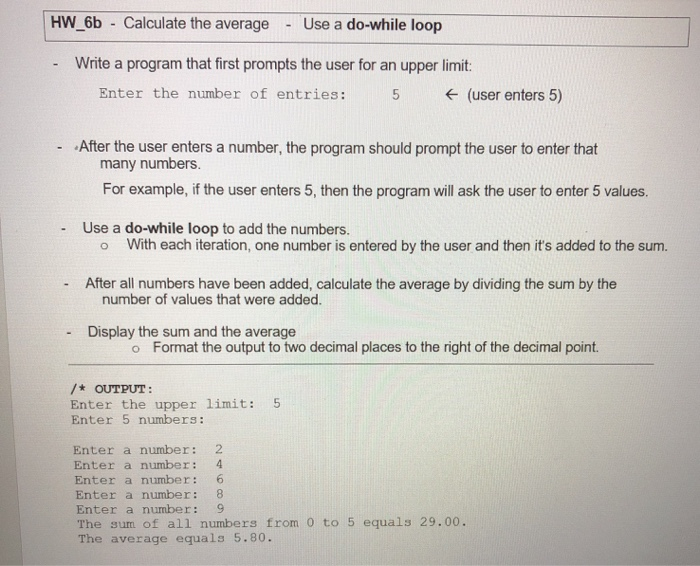need help!! c++ HW_6b - Calculate the average Use a do-while loop Write a program that first prompts the user for an upper limit: Enter the number of entries: 5 € (user enters 5) After the user enters a number, the program should prompt the user to enter that many numbers. For example, if the user enters 5, then the program will ask the user to enter 5 values. Use a do-while loop to add the numbers. o With each...

• ### Program C: Start by printing out your name, then your code should prompt the user to...

Program C: Start by printing out your name, then your code should prompt the user to enter integers using the following prompt: Enter some integers, end with a 0: Your code should: Level 1 Task: count the number of integers entered (not including the 0) Level 2 Task: produce the sum of all of the integers Level 3 Task: produce the sum of the even integers These calculated values need to be displayed as follows: There were ___ numbers read...

• ### 1. (sumFrom1.cpp) Write a program that will ask the user for a positive integer value. The...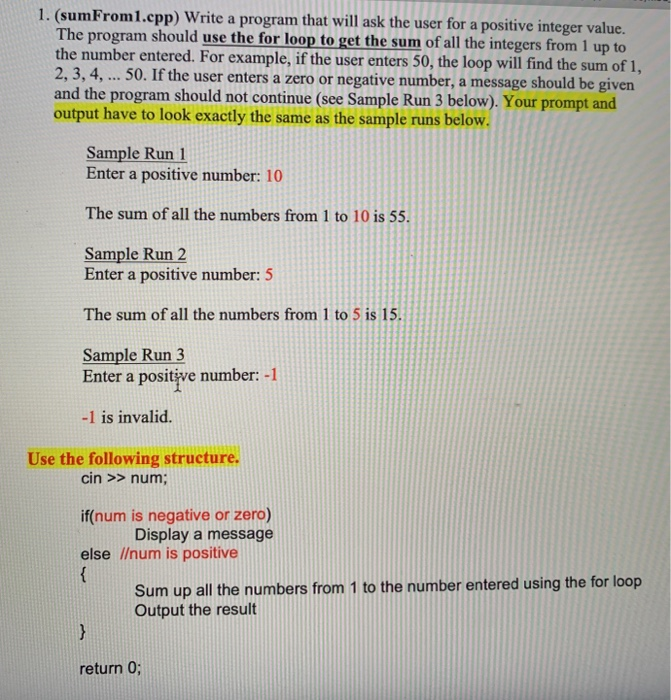1. (sumFrom1.cpp) Write a program that will ask the user for a positive integer value. The program should use the for loop to get the sum of all the integers from 1 up to the number entered. For example, if the user enters 50, the loop will find the sum of 1, 2, 3, 4, ... 50. If the user enters a zero or negative number, a message should be given and the program should not continue (see Sample Run...

• ### write the program in VBA b. Write a program that uses a For loop to perform...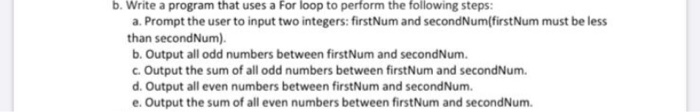write the program in VBA b. Write a program that uses a For loop to perform the following steps: a. Prompt the user to input two integers: firstNum and secondNum(firstNum must be less than secondNum). b. Output all odd numbers between firstNum and secondNum. c. Output the sum of all odd numbers between firstNum and secondNum. d. Output all even numbers between firstNum and second Num. e. Output the sum of all even numbers between firstNum and secondNum.

• ### 21 Write a program that asks the user to input the length and breadth of a...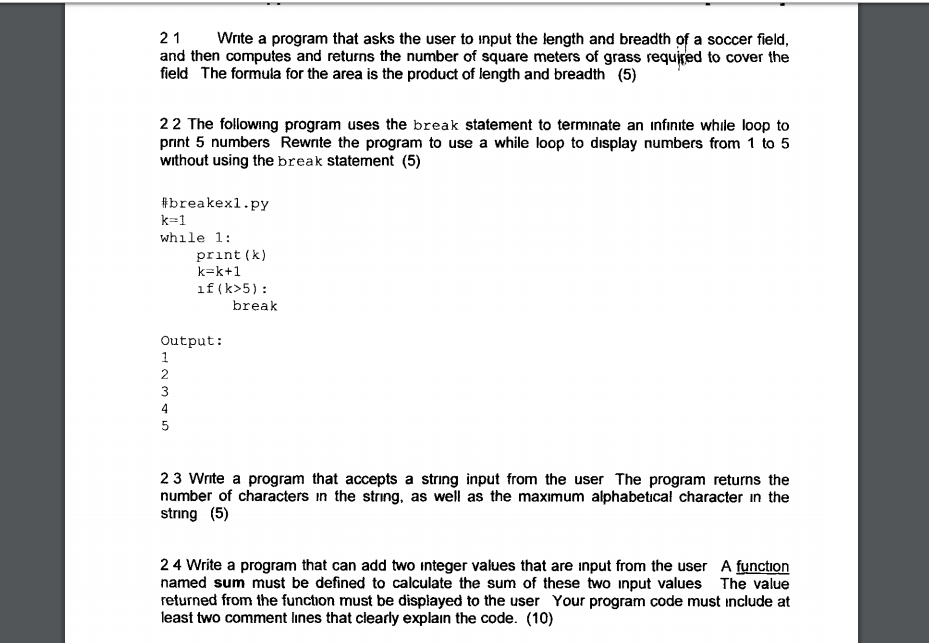21 Write a program that asks the user to input the length and breadth of a soccer field, and then computes and returns the number of square meters of grass required to cover the field The formula for the area is the product of length and breadth (5) 22 The following program uses the break statement to terminate an infinite while loop to print 5 numbers Rewrite the program to use a while loop to display numbers from 1 to...

• ### Using c++ ) You will write a program that will do the following: prompt the user...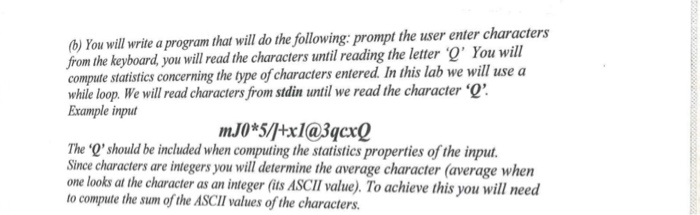Using c++ ) You will write a program that will do the following: prompt the user enter characters from the keyboard you will read the characters until reading the letter O' You will compute statistics concerning the type of characters entered. In this lab we will use a while loop. We will read characters from stdin until we read the character ' Example inpu mJ0*5/tx1@3qcx0 The 'Q'should be included when computing the statistics properties of the input. Since characters are...